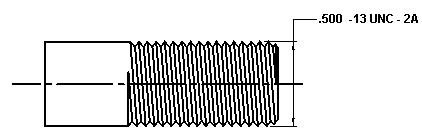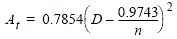Tensile Thread Stress Area Equation and Calculator less than 100 ksi

This calculator requires a java - enabled browser)

This calculator calculates the tensile stress area of a screw thread fastener. Use this calculator for materials less than 100,000 psi ultimate tensile strength.

The critical areas of stress of mating screw threads are:

Negative Tolerance numbers indicate interference.

This calculator will calculate the unknown for the given conditions. Two (2) inputs are required, See the application illustration at bottom of page.

The following are definitions for the elements:

At = tensile strength area of screw thread.

D = Basic major diameter, example; .500 for a 1/2 - 13 UNC - 2B Thread Engineering Data Chart

n = Number of threads per inchEquation:This equation provides an approximate result by extrapolation on the thread stress area of a fastener.This equation is adequate for design applications on engineering materials or less than 100 ksi ultimate strength.

Related:Membership Register | LoginHomeEngineering Book StoreEngineering ForumExcel App. DownloadsOnline Books & ManualsEngineering NewsEngineering VideosEngineering CalculatorsEngineering ToolboxGD&T Training Geometric Dimensioning TolerancingDFM DFA TrainingTraining Online EngineeringAdvertising CenterCopyright Notice